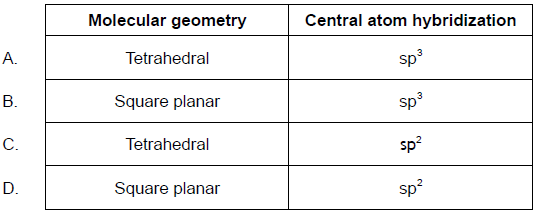# IB DP Chemistry Structure 2.4 From models to materials IB Style Question Bank HL Paper 1

## Question

What are the hybridizations of the atoms labelled 1, 2 and 3 in the molecule below?B

## Question

Which allotropes of carbon show $$s{p^2}$$ hybridization?

I.     Diamond

II.    Graphite

III.    $${C_{60}}$$ fullerene

A.     I and II only

B.     I and III only

C.     II and III only

D.     I, II and III

C

## Question

Which molecules have $${\text{s}}{{\text{p}}^{\text{2}}}$$ hybridization?

I.     $${{\text{C}}_{\text{2}}}{{\text{H}}_{\text{4}}}$$

II.     $${{\text{C}}_{\text{4}}}{{\text{H}}_{{\text{10}}}}$$

III.     $${{\text{C}}_{\text{6}}}{{\text{H}}_{\text{6}}}$$

A.     I and II only

B.     I and III only

C.     II and III only

D.     I, II and III

B

## Question

Which combination correctly describes the types of hybridization shown by the two carbon atoms labelled $$\alpha$$ and $$\beta$$ and the oxygen atom labelled $$\gamma$$ in the molecule of paracetamol shown below?A

## Examiners report

Although delocalization in amide is not covered in the syllabus, answer C was also accepted here as there is significant double bond character in the nitrogen to carbon (of the carboxamide group) bond. The question will be amended before publication.

## Question

Which combination describes the PH4+ ion?